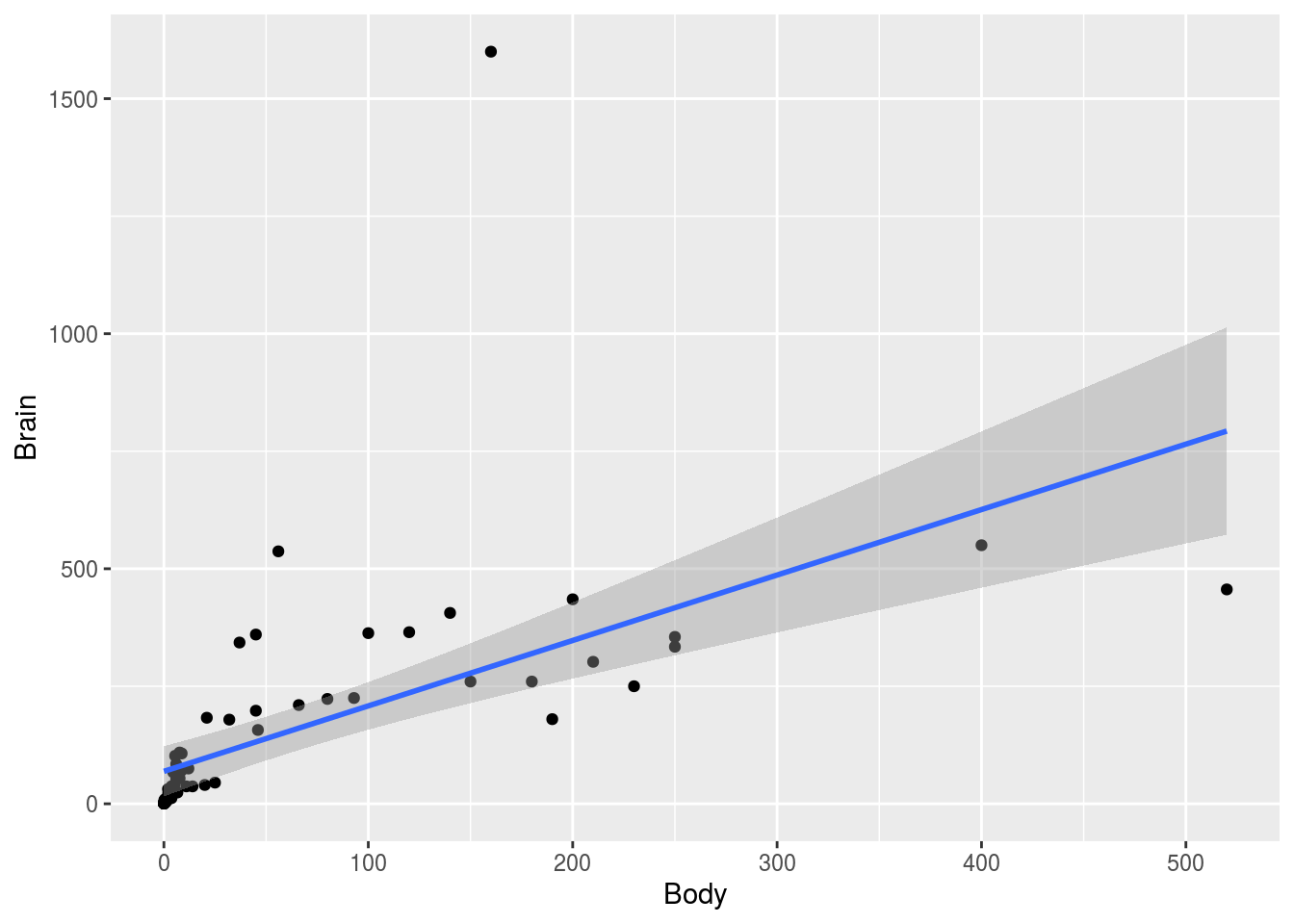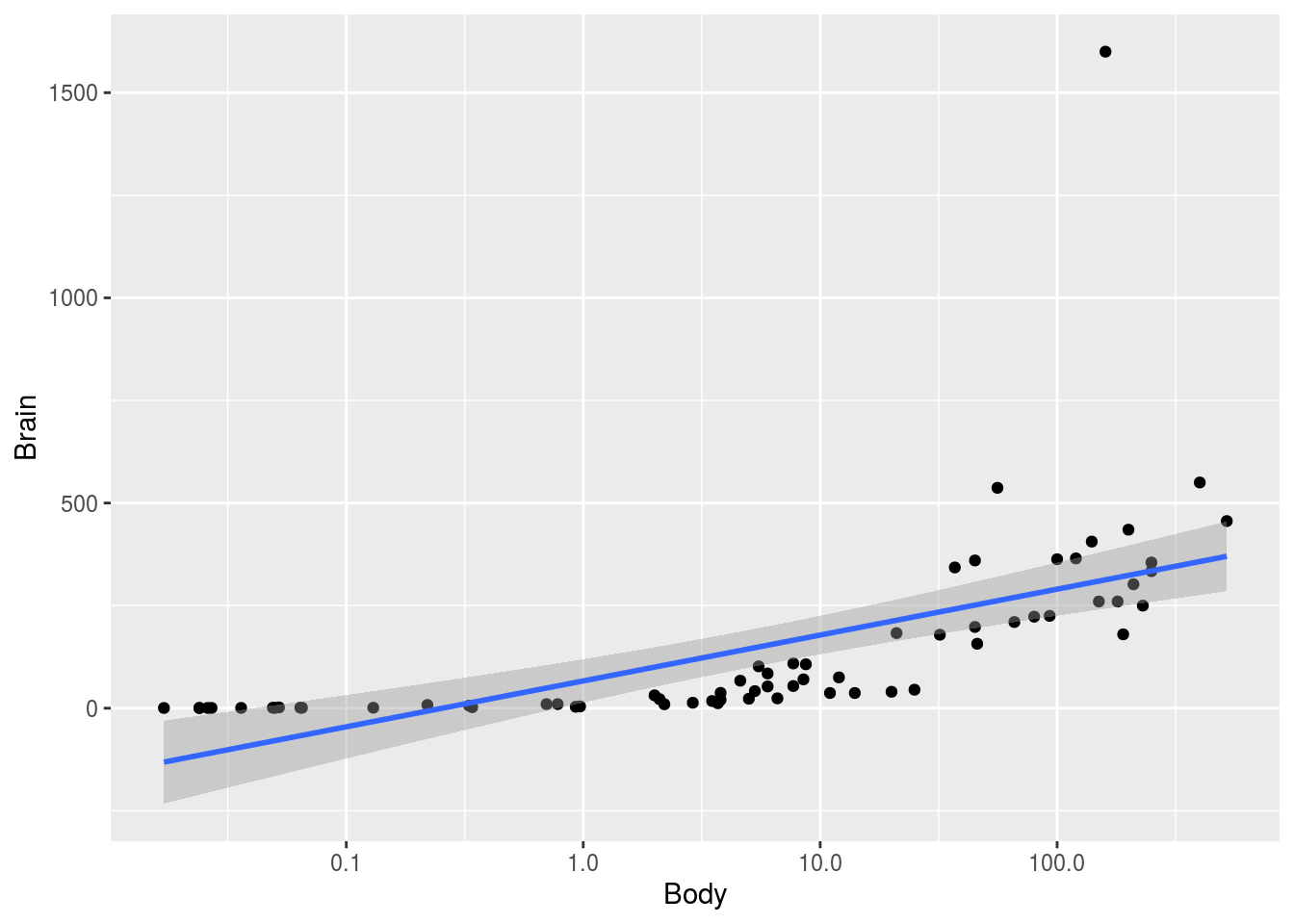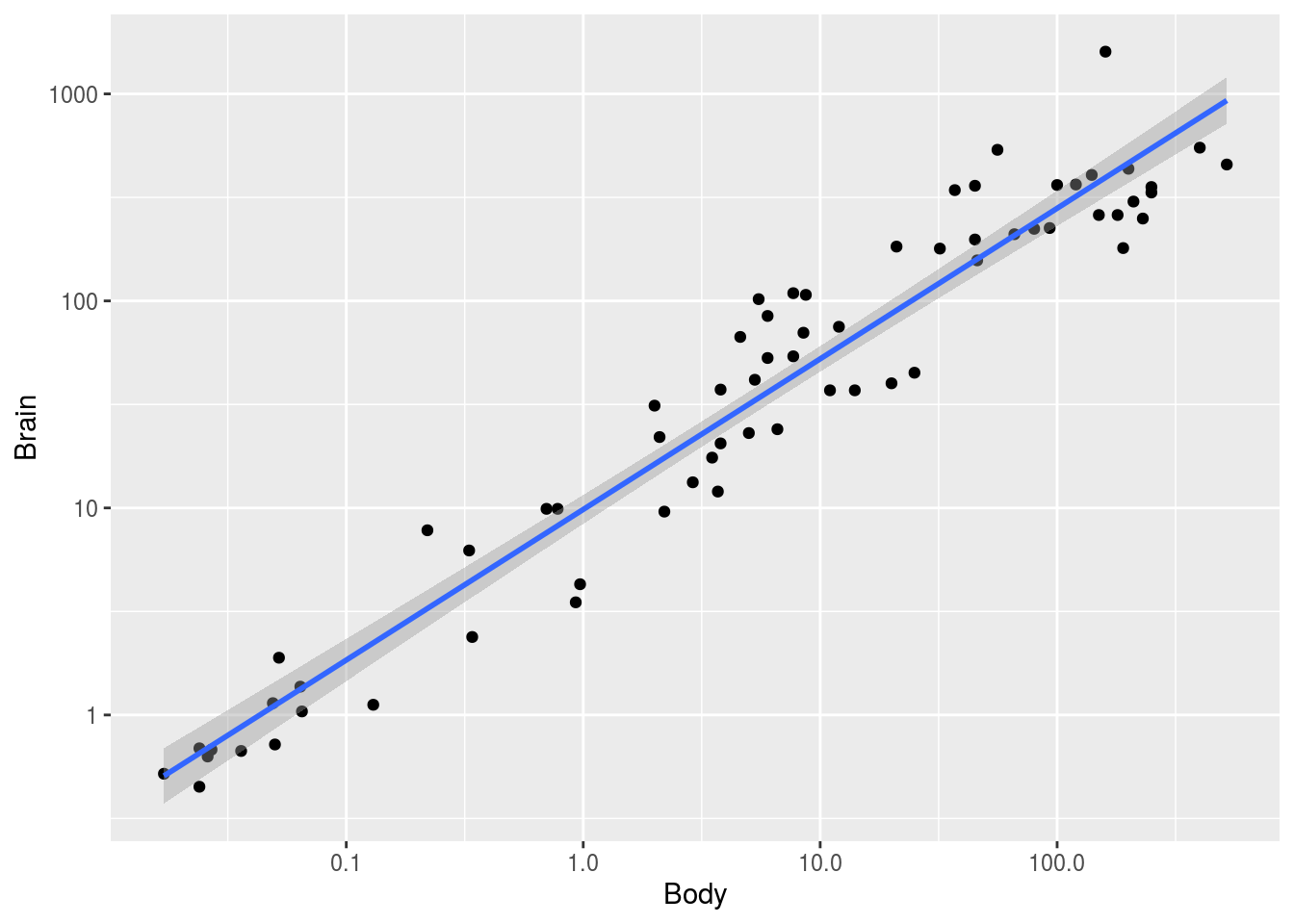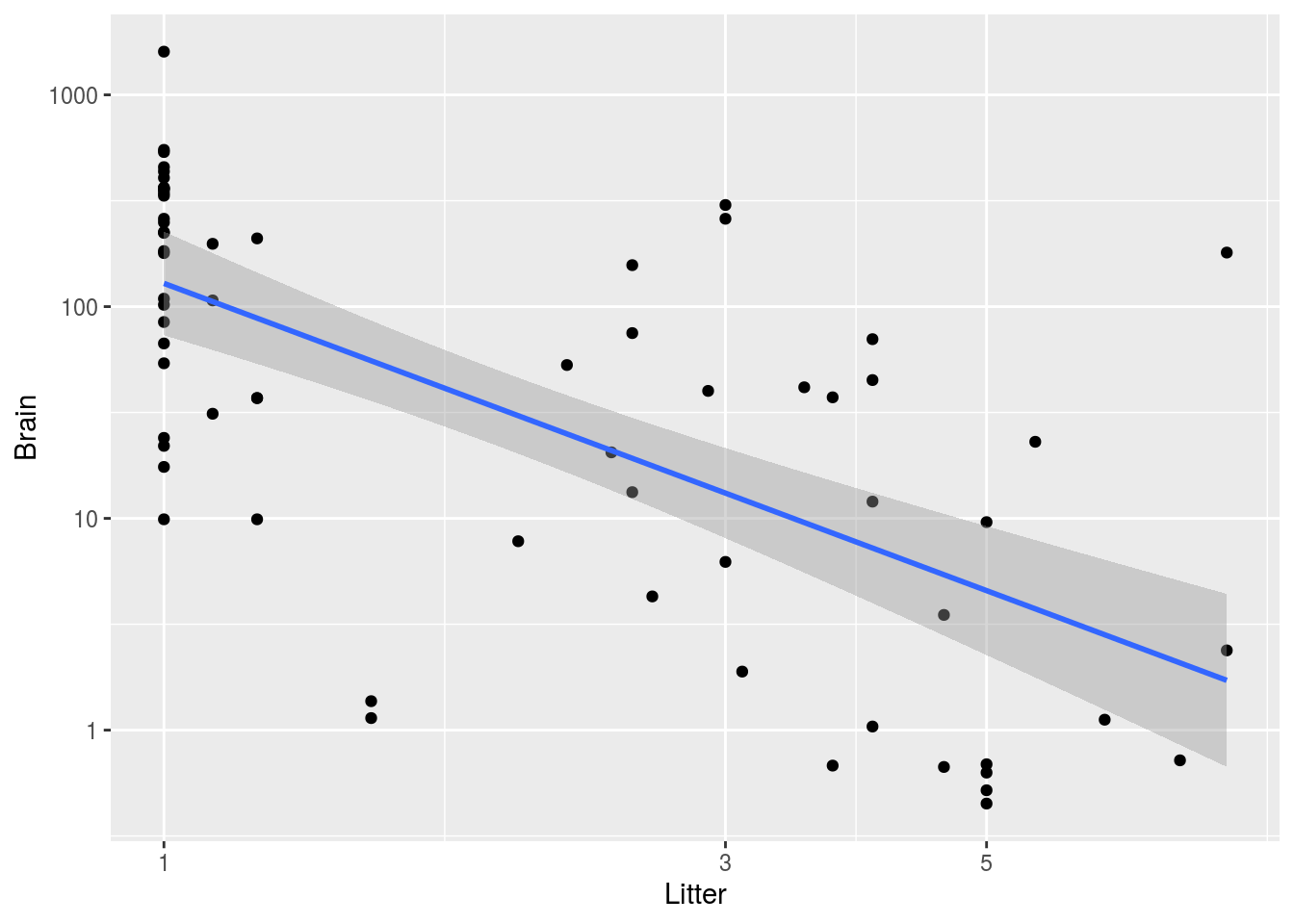### Question 1 (20 pts)

``brains <- read.csv("http://guerzhoy.princeton.edu/201s20/brains.csv")``

The dataset contains information about the weight of the brain, the weight of the body, the gestation period, and the litter size for a large number of mammals.

You should make a model that would do as good a job as possible (using the tools we use in the course) of predicting the weight of the brain of a new mammal, and estimate how well you’d be able to predict the weight of the brain of a new mammal. Follow the instructions below.

#### Question 1(a) (7 pts)

Split the dataset into training/test/validation sets. You may use a 70%/15%/15% Training/Test/Validation split. Explain why it is necessary to split the data into three sets. Explain why would you want the training set to be large. Explain why you would want the test and validation sets to be large.

#### Solution

``````idx <- sample(1:nrow(brains))
train.idx <- idx[1:round(0.7*length(idx))]
valid.idx <- idx[(round(0.7*length(idx)) + 1):(round(0.85*length(idx)))]
test.idx <-  idx[(round(0.85*length(idx)) + 1):length(idx)]

brains.train <- brains[train.idx, ]
brains.valid <- brains[valid.idx, ]
brains.test <- brains[test.idx, ]``````

We want the training set to be large to avoid overfitting. This will lead to more accurate predictions on new data.

We want the validation to be large so that we select the model that will generalize the best.

We want the test set to be large so that our estimate of how well we’ll do on new data will be more accurate.

#### Question 1(b) (6 pts)

Use at least three graphs to illustrate how you would use data visualization to make the best model possible. Use `ggplot`. Include the code you used in the R file that you submit. State what you see in the graphs and how you would use that.

#### Solution

``````library(tidyverse)
ggplot(data = brains.train) +
geom_point(mapping = aes(x = Body, y = Brain)) +
geom_smooth(mapping = aes(x = Body, y = Brain), method = "lm")``````It seems that a transformation might be appropriate:

``````ggplot(data = brains.train) +
geom_point(mapping = aes(x = Body, y = Brain)) +
geom_smooth(mapping = aes(x = Body, y = Brain), method = "lm") +
scale_x_log10()````````````ggplot(data = brains.train) +
geom_point(mapping = aes(x = Body, y = Brain)) +
geom_smooth(mapping = aes(x = Body, y = Brain), method = "lm") +
scale_x_log10() +
scale_y_log10()``````It seems the log(Brain) vs. log(Body) trend is linear.

``````ggplot(data = brains.train) +``````ggplot(data = brains.train) +